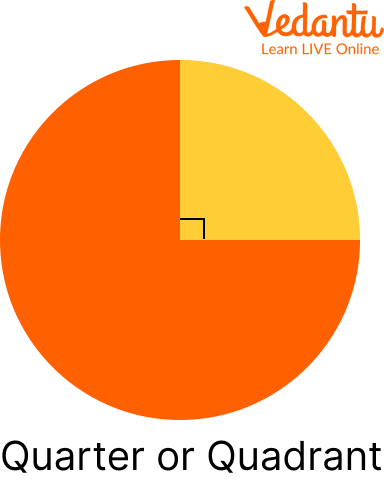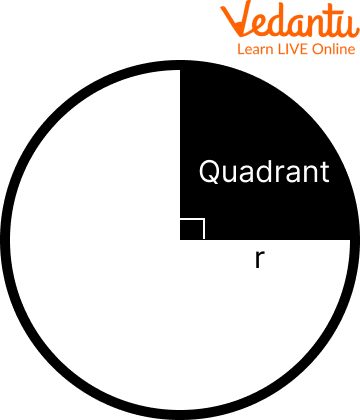Courses
Courses for Kids
Free study material
Free LIVE classes
More

# The Perimeter of a QuadrantLIVE
Join Vedantu’s FREE Mastercalss

## Introduction to the Perimeter of a Quadrant of a Circle

Geometry includes a crucial concept called perimeter finding that has many real-world applications. Quadrants can be seen in a variety of contexts, such as the outer shape of a baseball's "diamond" and a slice of cake.

Finding the perimeter of a form like this involves adding the lengths of the straight sections to the length of the curved section, which is the first step in determining the perimeter. Learning this method will provide you with a solid foundation for calculating the perimeters of several forms and expose you to an important tactic for handling issues similar to this in general.Quarter refers to a $\dfrac{1}{4}$ part of a thing or shape. A quarter circle is created by cutting a circle into four equal parts, or a semicircle into two equal sections.

## The Perimeter of a Circle

Perimeter of circle is nothing but the length of boundary of the circle which is also known as circumference of the circle. The entire perimeter of a circle is called the circumference and is given by $c=2 \pi r$, where $(c)$ means circumference and $(r)$ means radius.

## The Length of the Quadrant Curve

Since a quadrant is a quarter of a circle, to find the length of the curved part, take the circumference from the last step and divide it by 4. This helps clarify how the solution works, but you can also compute $0.5 \times \pi r$ by doing all of this in one step. The length of the curved portion is the outcome of this.## The Perimeter of a Quadrant

To find the perimeter of a quadrant we need length of curved part which can be found using the formula $0.5 \times \pi r$ and length of two straight lines which is equal to the radius as quadrant is combination of two radii and a curved part.

Therefore the formula can be written as:

The Perimeter of a Quadrant = $2r + 0.5 \times \pi r$

## The Area of ​​a Quadrant

The method used so far works for the length of an arc of a quarter circle, but a small change helps you find the area of ​​a quadrant with a very similar approach. the area of a circle is $\mathbf{a}=\pi \mathbf{r}^{2}$, so the area of a quadrant is $\mathbf{a}=\dfrac{\pi \mathbf{r}^{2}}{4}$, because it is one fourth the area of the circle.

### Solved Examples

Q 1 If you have a quadrant with r = 10, what will be its perimeter?

Sol: Put r = 10 in the perimeter of a quadrant formula

$p=0.5 \pi r+2 r$

After substituting the values, we get

$\Rightarrow p=0.5 \pi(10)+2(10)$

Now on solving it further, we get

$\Rightarrow p=5 \pi+20$

$\Rightarrow p=15.7+20$

$\Rightarrow p = 35.7$

Therefore, the perimeter will become 35.7 unit.

Q 2 What will be the perimeter of a quadrant if the diametre of the circle is 10 m.

Sol: The radius of the circle will be half of its diameter. Therefore,

$d=10 \mathrm{~m}$

$r=\dfrac{d}{2}$

$r=\dfrac{10}{2}$

$r=5 \mathrm{~m}$

Now the perimeter of the quadrant will be

$p=0.5 \pi r+2 r$

Now, on substituting the values, we get

$\Rightarrow p=0.5 \pi(5)+2(5)$

Solving further the above equation, we get

$\Rightarrow p=2.5 \pi+10$

$\Rightarrow p=7.85+10$

$\Rightarrow p=17.85 m$

Therefore, the perimeter of a quadrant if the diameter of the circle is 10 m will be 17.85m.

### Practice Questions:

Q 1 What will be the perimeter of a quadrant circle with radius r = 15m.

Ans: 53.55m

Q 2 If the diametre of a circle is 12m, find the perimeter of the quadrant of that circle.

Ans: 21.42m

Q 3 What will be the perimeter of a quadrant circle with radius r = 75m .

Ans: 267.75m

## Summary

The locus of all points that are equally distant from the centre is referred to as a circle. A circle is evenly divided into four pieces, or more specifically, four quadrants, by a set of two perpendicular lines. A quadrant is one-fourth of a circle's segment. The coordinate plane system's four quarters are referred to as quadrants. A quadrant is the name for one of the four divisions. A quadrant, or sector of 90 degrees, is what is referred to as a quarter of a circle in the context of circles. We have also discussed the perimeter of the quadrant.

Last updated date: 22nd Sep 2023
Total views: 94.5k
Views today: 1.94k

## FAQs on The Perimeter of a Quadrant

1. What is a quarter circle (quadrant)? What is the quadrant's perimeter formula?

A quarter circle is an area (or) section formed by two radii that are perpendicular to one another and one-fourth of a circle's circumference. This is also referred to as a circle's quadrant. For example, if a circle is divided into four equally sized pieces, each piece is a quarter circle or a quadrant. Two radii and one-fourth of a circle's circumference constitute a quarter-circle. Therefore $r+r+\dfrac{2 \pi r}{4}=2 r+\dfrac{\pi r}{2}$ is the perimeter of a quarter circle of radius " $r$ ".

2. How will you find the perimeter of a quadrant of a circle formula if the radius is not given?

If radius $(r)$ is not given but the length of the curved section is given, you can use the circumference formula to find $(r)$. since $c=2 \pi r$, this means $r=\dfrac{c}{2 \pi}$. If you have the measurement for a quarter arch, simply multiply that by 4 to find $(c)$, and proceed to find $(r)$. Once you have found $(r)$, add $(2 r)$ to the length of the curved section to find the total perimeter.

3. How is a perimeter of a rectangle determined?

The perimeter of the rectangle is equal to the sum of the lengths of its four sides. It is simple to do this since there are two sets of equal side length; all that is needed is to sum the length and breadth and multiply the result by two.